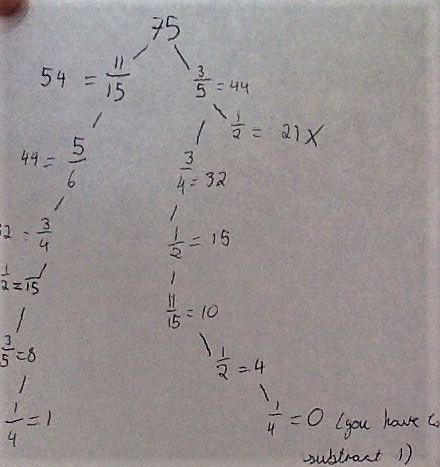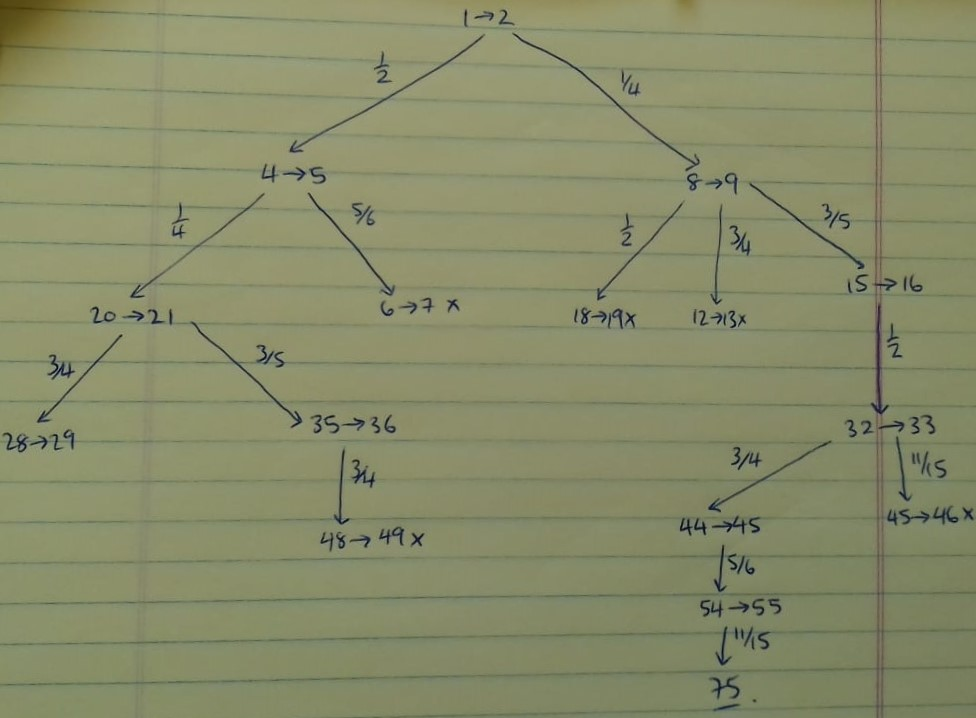#### You may also like### Arithmagons Poster

Arithmagons Poster### Harmonic Triangles Poster

Harmonic Triangles Poster### Squares in Rectangles Poster

Squares In Rectangles Poster

# Peaches Today, Peaches Tomorrow Poster

##### Age 11 to 16Challenge Level

$$\frac{11}{15},\frac56,\frac34, \frac12, \frac35, \frac14$$

Why?

Starting from the beginning

Start with a denominator which can divide 75 without a remainder. The options are $\frac{11}{15}$ or $\frac35.$ Here you have to choose one and see if it works. This tree shows all of the possibilities:Starting from the end

On the last day, the fraction he wants to keep needs to be $\frac12$ or $\frac14$, or a fraction with the numerator as 2. This is because the number of peaches has to be 2 (because he eats 1), but if the numerator is larger than 2, then there will be more than 2 peaches.

This tree shows the possibilities working from the end: